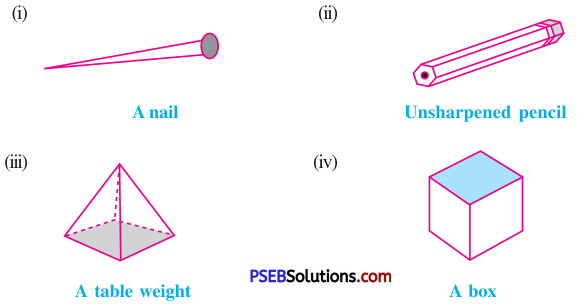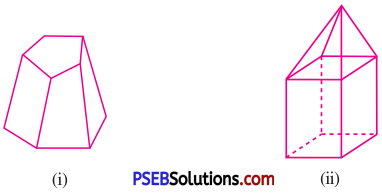# PSEB 8th Class Maths Solutions Chapter 10 Visualising Solid Shapes Ex 10.3

Punjab State Board PSEB 8th Class Maths Book Solutions Chapter 10 Visualising Solid Shapes Ex 10.3 Textbook Exercise Questions and Answers.

## PSEB Solutions for Class 8 Maths Chapter 10 Visualising Solid Shapes Ex 10.3

1. Can a polyhedron have for its faces:

Question (i)
3 triangles?
Solution:
[Note: Polyhedron is a solid, which is made by polygonal regions and these polygonal regions are called its faces.]
No, a polyhedron cannot have 3 triangles for its faces because it have atleast 4 faces.Question (ii)
4 triangles?
Solution:
Yes, a polyhedron can have 4 triangles for its faces as triangular pyramid.

Question (iii)
a square and four triangles?
Solution:
Yes, a polyhedron can have a square and four triangles for its faces as a pyramid with square base.

2 Is it possible to have a polyhedron with any given number of faces? (Hint: Think of a pyramid.)
Solution:
Yes, it can be possible only if the number of faces is greater than or equal to 4, because a polyhedron has atleast 4 faces.3. Which are prisms among the following?Solution:
Note: A prism is a polyhedron whose base and top are congruent polygons and lateral faces are rectangles.
(i) No, a nail is not a prism because its base and top are not congruent polygons.
(ii) Yes, an unsharpened pencil is a prism because its base and top are congruent polygons and faces are rectangles.
(iii) No, a table weight is not a prism because its top and base are not congruent polygons.
(iv) Yes, box is a prism because its base and top are congruent polygons and lateral faces are rectangles.

4.

Question (i)
How are prisms and cylinders alike?
Solution:
Top and base of a prism and of a cylinder are congruent and parallel to each other and a prism becomes a cylinder, if the number of sides of its base becomes larger and larger.Question (ii)
How are pyramids and cones alike?
Solution:
The pyramids and cones are alike because their lateral faces meet at a vertex. Also, a pyramid becomes a cone if the number of sides of its base becomes larger and larger.

5. Is a square prism same as a cube? Explain.
Solution:
No, a square prism is not same as a cube. It can be a cuboid also.

6. Verify Euler’s formula for these solids:Solution:
(i) For figure (i)
F = 7, V= 10 and E = 15
∴ F + V = 7 + 10 = 17
Now, F + V – E = 17 – 15 = 2
Thus, F + V – E = 2
Hence, Euler’s formula is verified.

(ii) For figure (ii)
F = 9, V = 9 and E = 16
∴ F + V = 9 + 9 = 18
Now, F + V – E = 18 – 16 = 2
Thus, F + V – E = 2
Hence, Euler’s formula is verified.7. Using Euler’s formula find the unknown:

 (i) (ii) (iii) Faces ? 5 20 Vertices 6 ? 12 Edges 12 9 ?

Solution:
(i) Here, F = ?, V = 6 and E = 12
Now, F + V – E = 2 (∵ Euler’s formula)
∴ F + 6 – 12 = 2
∴ F – 6 = 2
∴ F = 2 + 6
∴ F = 8

(ii) Here, F = 5, V = ? and E = 9
Now, F + V – E = 2 (∵ Euler’s formula)
∴ 5 + V – 9 = 2
∴ V – 4 = 2
∴ V = 2 + 4
∴ V = 6

(iii) Here, F = 20, V = 12 and E = ?
Now, F + V – E = 2 (∵ Euler’s formula)
∴ 20 + 12 – E = 2
∴ 32 – E = 2
∴ – E = 2 – 32
∴ – E = – 30
∴ E = 308. Can a polyhedron have 10 faces, 20 edges and 15 vertices?
Solution:
Here, F = 10, E = 20 and V = 15
By Euler’s formula, F + V – E = 2
LHS = F + V – E
= 10 + 15 – 20
= 25 – 20 = 5
Thus, F + V – E ≠ 2
Hence, such a polyhedron is not possible.